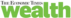# How to calculate your investment returns using this MS-Excel tool

## Geometric mean scores over arithmetic mean as it takes into account the effect of volatility. The GEOMEAN function helps you to calculate tedious geometric mean returns in a few clicks. Use right variant of average return for effective planning.

, ET Bureau|
Updated: Dec 16, 2019, 11.43 AM IST
Getty ImagesThe return generated by Sensex yesterday has an influence on the returns generated today.
The average rate of returns plays a critical role in personal finance calculations. For making assumptions, the historical average return is often used as an initial basis. If the assumed average return is over-estimated, it could ruin the whole long-term investment planning.

For example, if an asset’s average future return is over-estimated at 12% per annum instead of the actual return of 10% per annum, one needs to invest only Rs 10,109 every month to achieve a goal of accumulating Rs 1 crore in 20 years. However, the actual requirement to meet the goal at 10% is Rs 13,169. Thus, in case of over-estimating returns, the corpus will fall short by Rs 23.24 lakh at the end of the tenure.

Therefore, the usage of the correct variant of the average return is critical for effective financial planning. One of the easiest and widely known measures of calculating returns is the arithmetic average (arithmetic mean or AM). In terms of investments, the AM of an asset is calculated by adding the returns over a given period of time and dividing by the total number of the time periods. Looking at the BSE Sensex, the index has delivered 27.9%, 5.9% and 13.1% returns in 2017, 2018 and 2019 respectively. Therefore, the AM will be 46.9% (27.9% + 5.9% + 13.1%) divided by 3 (total number of years), which equals 15.6%.

Although simple to calculate, AM is useful when such returns are independent. However, financial data exhibits serial correlation where the returns generated by an asset in any defined interval (daily, weekly, monthly or yearly) get influenced by the returns generated by the asset in the previously defined intervals. For example, the return generated by Sensex yesterday has an influence on the returns generated today.

Use right variant of average return for effective planning
GEOMEAN function helps you calculate tedious geometric mean returns in a few clicks.
Screenshot 1

Due to such serial correlation, AM may be misleading at times. In the Sensex example, the value of Rs 1 lakh invested in 2017 will grow to Rs 1,54,480 in 2019, using the arithmetic return of 15.6% compounded annually. However, if we use the long calculation method, Rs 1 lakh will become Rs 1,27,900 (1,00,000 X (1+27.9%))in 2017, Rs 1,35,446 (127,900(1+5.9%)) in 2018 and Rs 1,53,190 ((1,35,446 X (1+13.1%)] in 2019. That means, the usage of AM has overstated the value by Rs 1,290.

To take care of such anomalies, the geometric average (geometric mean or GM) should be preferred over the arithmetic mean. GM is calculated by multiplying all the periodic returns and taking the root of the number of such durations. For the above example, the GM is calculated as [{(1+27.9%) X (1+5.9%) X (1+13.1%)}^(1/3)-1], which equals the actual return of 15.28%. Here, 1 is added to the returns to take care of any negative numbers (or negative returns). As only three-year period is considered in the above example, the multiplicative value of the returns is raised to the root of 3.

Using the GM of 15.28% compounded annually for 3 years, the value of Rs 1 lakh turns into Rs 1,53,190 [1,00,000 X (1+15.28%)^3], which is accurate and also derived using the long calculation method.

AM also loses its effectiveness due to the integrated volatility in the markets and the compounding effect. AM will always be higher than GM, unless there is zero volatility. Generally, higher the volatility, higher will be the difference between the two. As the assets in the equity, debt, and currency markets exhibit recurrent volatility, GM is more appropriate for calculations.

The above example has only 3-year values, however, the data may run into longer time periods making GM calculation complex. However, it can be calculated easily using MS Excel’s GEOMEAN function. On the other hand, the AM can be worked out using the AVERAGE function. The usage of GEOMEAN is demonstrated in screenshot-1 and the AVERAGE function in screenshot-2. To arrive at the GM, 1 needs to be subtracted from the formula as the same has been added to the returns (to take care of any negative returns) that were used as an input in the GEOMEAN function (column C in screenshot-1).

It is worth mentioning that compound annual growth rate(CAGR) and the GM are same and generate similar figures. However, both differ in their computation formulas and data requirements. While CAGR works on the start and the end values and the number of periodic intervals, GM works on time series data of asset returns.

Focus on investment and exports to revive investment, economic cycles: Kiran Mazumdar Shaw

Pine Labs announces investment by Mastercard

What are the dual advantages of investing in ELSS?

Tax Saving Investment Options for salaried

Bharat Bond ETF: Why to invest and who should invest?

Commenting feature is disabled in your country/region.